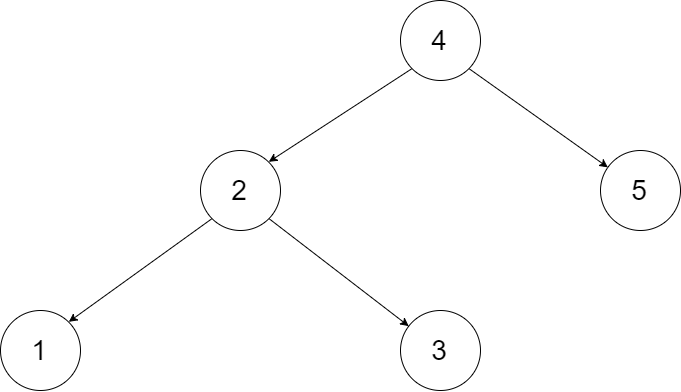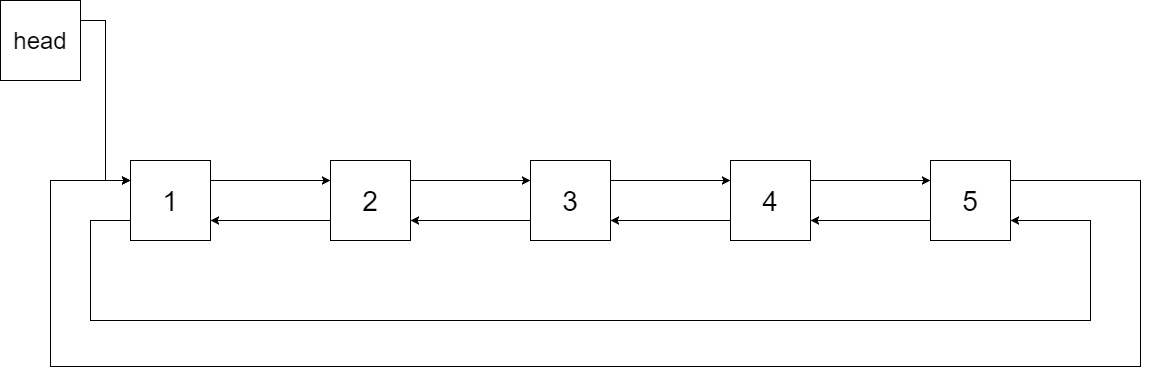# 剑指 Offer 36. 二叉搜索树与双向链表

### 题目### 题解

``````public Node treeToDoublyList(Node root) {
// left表示头结点 right表示尾节点
Node result = new Node(0);
// 使用中序遍历
// 第一个节点为头结点 最后一个节点为尾节点
Consumer<Node> recursion = new Consumer<Node>() {
@Override
public void accept(Node node) {
if (node == null) {
return;
}
this.accept(node.left);
if (result.right == null) {
// 设置头结点位置
result.left = node;
} else {
// 双指针当前节点和尾节点
result.right.right = node;
node.left = result.right;
}
// 设置最后访问的节点 即尾节点
result.right = node;
this.accept(node.right);
}
};

recursion.accept(root);
// 头、尾节点指针
if (null != result.left) {
result.left.left = result.right;
result.right.right = result.left;
}

return result.left;
}
``````